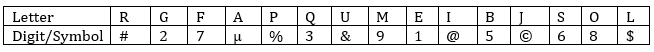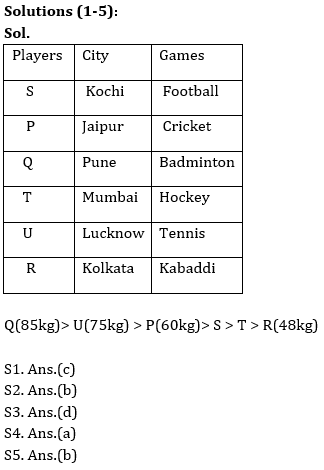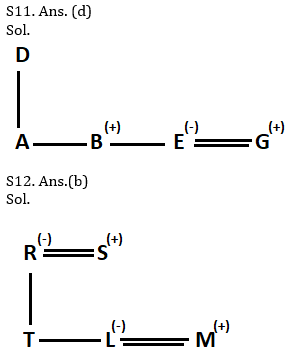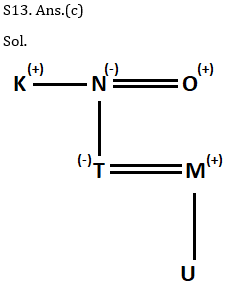Latest Banking jobs   »   Reasoning Ability Quiz For RRB PO...   »   Reasoning Ability Quiz For RRB PO...

# Reasoning Ability Quiz For RRB PO Mains 2021- 23rd September

Directions (1-5): Read the given information carefully and answer the given questions.
Six players are belonging to different cities. All of them Plays different games. Each player has different weight. S’s weight is more than only 2 persons and he belong to Kochi. The one who belongs to Jaipur has weight which is more than T’s weight but less than U’s weight. The one who likes Cricket, belongs to Jaipur. R doesn’t like Cricket. P’s weight is more than R but less than Q. Q belongs to Pune and T likes Hockey. The one, who is heaviest, has 85kg weight and likes Badminton. T is not the person who has lowest weight. U doesn’t like Badminton. The one who likes Kabaddi doesn’t belong to Kochi and he is not the person who has second highest weight. P’s weight is 60kg. The one who has 75kg weight and likes Tennis and belongs to Lucknow. R doesn’t belong to Mumbai. The one who has lowest weight is 48kg. The one who belongs to Kolkata does not play football.

Q1. Who among the following persons belongs to Kolkata?
(a)S
(b)Q
(c) R
(d) P
(e)None of these

Q2. Who among the following person is 75kg?
(a)Q
(b)U
(c)R
(d)P
(e)None of these

Q3. How many persons are lighter than Q?
(a)None
(b)Two
(c)Four
(d)Five
(e)Three

Q4. The one who plays badminton belongs to which city?
(a) Pune
(b)Jaipur
(c)Mumbai
(d)Lucknow
(e)None of these

Q5. The person who is lightest in the group plays which of the following games?
(a)Cricket
(c) Tennis
(d)Football
(e)None of these

Directions (6-10): In each of the following below is given a group of letters followed by four combinations of digits/symbols numbered (a), (b), (c) and (d). You have to find out which of the combinations correctly represents the group of letters based on the following coding system and mark the number of that combination as the answer. If none of the four combinations correctly represents the group of letters, mark (e), i.e. ‘None of these’, as the answer-

Note: More than one condition may apply.Conditions:
(i) If first letter is vowel and last letter is consonant then both are coded with the code of the consonant.
(ii) If both the 2nd and the last letter is vowel, then their codes are to be interchanged.
(iii) If the second letter is a consonant and the 2nd last letter is a vowel, both are to be coded as the code for the vowel.
(iv) If both 1st and fifth letter is consonant then both are coded as the code of third letter.
(v) If only one condition is applied among the above given, then the code of first letter is interchanged with code of second letter and third letter code interchanged with 4th letter and so on after that applied condition.

Q6. URBSAQ
(a) 3#65#3
(b) 3µ56µ3
(c) &56\$33
(d) &µ65µ&
(e) None of these

Q7. LIFPRE
(a) 7177%@
(b) 771%7@
(c) 717%@7
(d) 717%7@
(e) None of these

Q8. EUMRJA
(e) None of these

Q9. MJGLBF
(a) %3%6%7
(b) 36%%7@
(c) 3#6%7@
(d) 3%6%7#
(e) None of these

Q10. LRQBJS
(a) %53328
(b) #33536
(c) #35363
(d) @3567@
(e) None of these

Directions (11-13): In the following questions, the symbols &, @, * , \$, % and © are used with the following meanings as illustrated below. Study the following information and answer the given questions:
P@Q- P is the child of Q
P©Q- P is the parent of Q
P%Q- P is father-in-law of Q
P&Q- P is brother-in-law of Q
P\$Q- P is brother of Q
P*Q- P is wife of Q

Q11. If the expression ‘A@D©B\$E*G’ is true, then how G is related to D?
(a) Father-in-law
(b) Brother
(c) Son
(d) Son-in-law
(e) Cannot be determined

Q12. If the expression ‘L*M&T@R*S©L’ is true, then which of the following is true?
(a) L is son of R
(b) R is mother-in-law of M
(c) T is daughter of S
(d) S is mother-in-law of M
(e) None is true

Q13. If the expression ‘K\$N©T@O%M©U’ is true, the which of the following is not true?(O has only one daughter).
(a) U is grandchild of O
(b) K is uncle of T
(c) M is mother of U
(d) N is grandmother of U
(e) All are true

Q14. If in the number 472561839, 2 is multiply to each of the digit which is less than five and 2 is subtracted from each of the digit which is greater than four then all digits are arranged in ascending order from left to right. What is the resultant of the multiplication of the digit which is third from the left end and the digit which is fourth from the right end in new arrangement?
(a) 12
(b) 24
(c) 32
(d) 20
(e) 27

Q15. If all the vowels of the word ‘SURVEILLANCE’ are replaced by its succeeding letter according to the English alphabet and all the consonant are replaced with their previous letter according to the English alphabet and then all the letters are arranged in the alphabetical order the how many letter are there between the third letter from the left and fourth letter from the right in the English alphabetic series?
(a) 5
(b) 12
(c) 11
(d)10
(e) 9

SolutionsS6. Ans(b)
Sol. Condition (i) and (iii) is applied

S7. Ans(d)
Sol. Condition (ii) and (iv) is applied

S8. Ans(a)
Sol. Condition (ii) and (v) is applied

S9. Ans(e)
Sol. Condition (iv) and (v) is applied

S10. Ans(c)
Sol. Condition (iv) and (v) is appliedS14. Ans.(b)
Sol. Given number- 472561839
After applied given condition- 854342667
Arrange in ascending order- 234456678

S15. Ans.(d)
Sol. Given word- SURVEILLANCE
After applied given condition- RVQUFJKKBMBF
Arranged in alphabetical order- BBFFJKKMQRUV

Click Here to Register for Bank Exams 2021 Preparation Material#### Congratulations!Download Hindu Review of October 2021: Free PDF